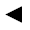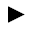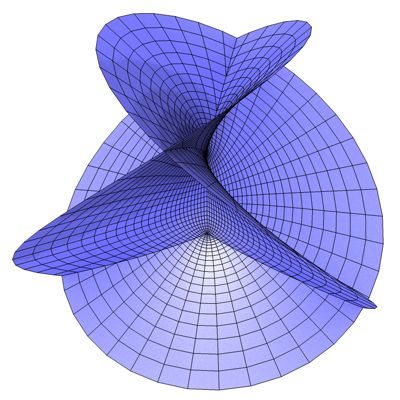[back]

## 3.28. Henneberg's Surface

[next]The Henneberg's surface [ 3,8,11 ] is represented by the following equations.

 x = 2 cos(v) sinh(u) - 0.667 cos(3 v) sinh(3 u) 3-99 y = 2 sin(v) sinh(u) + 0.667 sin(3 v) sinh(3 u) 3-100 z = 2 cos(2v) cosh(2u) 3-101

To represent the area, the two parameters u and v can assume the following values ​​(domain of definition), for example.

 u is an element from the set of numbers [-1, 1] v is an element from the number set [-pi/2, pi/2]

Henneberg's surface is a half-open figure, so the definition range of u can be changed with the plugin.Fig. 32